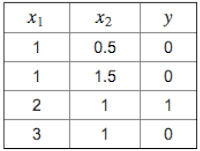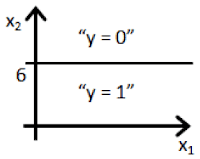# ▸ Logistic Regression :

Recommended Machine Learning Courses:

1. Suppose that you have trained a logistic regression classifier, and it outputs on a new example a prediction = 0.2. This means (check all that apply):
• Our estimate for P(y = 1|x; θ) is 0.8.
h(x) gives P(y=1|x; θ), not 1 - P(y=1|x; θ)
• Our estimate for P(y = 0|x; θ) is 0.8.
Since we must have P(y=0|x;θ) = 1 - P(y=1|x; θ), the former is
1 - 0.2 = 0.8.
• Our estimate for P(y = 1|x; θ) is 0.2.
h(x) is precisely P(y=1|x; θ), so each is 0.2.
• Our estimate for P(y = 0|x; θ) is 0.2.
h(x) is P(y=1|x; θ), not P(y=0|x; θ)

1. Suppose you have the following training set, and fit a logistic regression classifier .Which of the following are true? Check all that apply.
• Adding polynomial features (e.g., instead using ) could increase how well we can fit the training data.
• At the optimal value of θ (e.g., found by fminunc), we will have J(θ) ≥ 0.
• Adding polynomial features (e.g., instead using ) would increase J(θ) because we are now summing over more terms.
• If we train gradient descent for enough iterations, for some examples in the training set it is possible to obtain .

1. For logistic regression, the gradient is given by . Which of these is a correct gradient descent update for logistic regression with a learning rate of ? Check all that apply.
• (simultaneously update for all j).
• .
• (simultaneously update for all j).
• (simultaneously update for all j).

1. Which of the following statements are true? Check all that apply.
• The one-vs-all technique allows you to use logistic regression for problems in which each comes from a fixed, discrete set of values.
If each is one of k different values, we can give a label to each and use one-vs-all as described in the lecture.
• For logistic regression, sometimes gradient descent will converge to a local minimum (and fail to find the global minimum). This is the reason we prefer more advanced optimization algorithms such as fminunc (conjugate gradient/BFGS/L-BFGS/etc).
The cost function for logistic regression is convex, so gradient descent will always converge to the global minimum. We still might use a more advanced optimisation algorithm since they can be faster and don’t require you to select a learning rate.
• The cost function for logistic regression trained with examples is always greater than or equal to zero.
The cost for any example is always since it is the negative log of a quantity less than one. The cost function is a summation over the cost for each sample, so the cost function itself must be greater than or equal to zero.
• Since we train one classifier when there are two classes, we train two classifiers when there are three classes (and we do one-vs-all classification).
We will need 3 classfiers. One-for-each class.

### Check-out our free tutorials on IOT (Internet of Things):

1. Suppose you train a logistic classifier . Suppose , , . Which of the following figures represents the decision boundary found by your classifier?
• Figure:In this figure, we transition from negative to positive when x1 goes from left of 6 to right of 6 which is true for the given values of θ.
• Figure:• Figure:• Figure:&
Click here to see more codes for Raspberry Pi 3 and similar Family.
&
Click here to see more codes for NodeMCU ESP8266 and similar Family.
&
Click here to see more codes for Arduino Mega (ATMega 2560) and similar Family.

Feel free to ask doubts in the comment section. I will try my best to answer it.
If you find this helpful by any mean like, comment and share the post.
This is the simplest way to encourage me to keep doing such work.

Thanks & Regards,
- APDaga DumpBox

1.Fifth question is wrong

1.Ok. What do you think the correct answer is??

2.3.Thanks for the response.But simply Not possible.
If you substitute to value of theta1 & theta2 in the equation given in the question, you will get x1=6. If you plot the graph x1=6 line, that line will always be parallel to x2 axis.

4.no ur answer is wrong ..it will be D..THANK ME LATER

5.6.Hi @Akshay, your answer is correct. I took the rest, and the reason why shivam and ravi say its D is because the question is different. (I got the same question that they got and the answer is D). The question reverses theta 1 = 0 and theta 2 = 1, in which case D is correct. They read the question wrong

7.Thank you Sneha.
This will help many others.

8.is there a discord server for discussion?? or asking questions

2.2nd question has stupid options which dont make much sense without knowing formula

3.theta =
6
-1
0

y = 1 if 6 + (-1(x1) + (0*x2) => GE(greaterThanEqualTo) 0
6 - x1 => = 0
-x1 => -6
x1 <= (lessThanEqualto) <= 6
therefore the decision boundary is a vertical line where x1=6 and everything to the left
of that denotes y = 1 , while everything to the right denotes y = 0

you don't have to copy somebody's answer it is in the course notes.

1.Thanks for the detailed explanation.

So For other viewers, to avoid the confusion, The correct answer for Que 5 is "A" only.